• 算法作业4 最优二分检索树的实现。 问题描述：给定n个标识符，a1 ...记由ai+1,ai+2,…,ajEi,Ei+1,…,Ej构成的最优二分检索树的预期成本为C(i, j)，设二分检索树T以ak为根。则有 COST(L) = C(0,k-1
算法作业4 最优二分检索树的实现。

问题描述：给定n个标识符，a1<a2<……<an,已知成功检索的概率p[i],不成功检索的概率q[i]，(0<=i<=n)，构造一颗检索成本最小的二分检索树。
算法思想：采用动态规划的方法，如果ak为最优二分检索树的跟，则其左子树也是一棵最优二分检索树，同理，右子树也是一棵最优二分检索树。

记由ai+1,ai+2,…,aj和Ei,Ei+1,…,Ej构成的最优二分检索树的预期成本为C(i, j)，设二分检索树T以ak为根。则有

COST(L) = C(0,k-1)
COST(R) = C(k,n)
这里做右子树的计算COST都是以其子树的根为第一级来计算的，所以会有成本差额w(0,k)和w(k,n)
c(0,n)=min{c(0,k)+c(k+1,n)+w(0,n)}
w(0,n)=p[k]+w(0,k-1)+w(k,n).

采用向前递推过程：
首先计算所有j-i=1的c(i,j)；
然后依次计算j-i=2,3,……n的c(i,j)；
c(0,n)等于最优二分检索树的成本。
初始值c(i,i)=0,w(i,i)=q(i),0<=i<=n,
在计算C(i, j)的过程中，记下使之取得最小值的k值，即树Tij的根，记为R(i, j)。
根据R(i,j)推导树的形态。

#include <stdio.h>
#include <stdlib.h>
#include <string.h>
float *p,*q;
//float p={-1,3,3,1,1};
//float q={2,3,1,1,1};
float **c,**w;
int **R;
int n;
//int n=4;
char *a;
//char a={{'d','o'},{'i','f'},{'r','e','a','d'},{'w','h','i','l','e'}};
typedef struct BiTNode{
int num;
BiTNode* Lc;
BiTNode* Rc;
}BiTNode, *BiTree;

//录入标识符集和成功检索概率以及不成功检索概率
void input()
{
int i;
printf("请输入字符个数：");
scanf("%d",&n);
p=(float *)malloc(sizeof(float)*(n+1));
q=(float *)malloc(sizeof(float)*(n+1));

printf("请键入字符集：\n");
for(i=0;i<n;i++)
{
printf("a[%d]=",i);
a[i]=(char *)malloc(sizeof(char)*10);
scanf("%s",a[i]);
}

p=-1;
for(i=1;i<=n;i++)//输入各字符检索成功的概率
{
printf("p[%d]=",i);
scanf("%f",&p[i]);
}

for(i=0;i<=n;i++) //输入检索不成功的概率
{
printf("q[%d]=",i);
scanf("%f",&q[i]);
}
}

void OBST( )
{

c=(float **)malloc(sizeof(float)*(n+1));
w=(float **)malloc(sizeof(float)*(n+1));
R=(int **)malloc(sizeof(int)*(n+1));
int i,j,m,k,l;
float tmp,tmp1;
for(i=0;i<=n;i++)
{
c[i]=(float *)malloc(sizeof(float)*(n+1));
memset(c[i],0,sizeof(float)*(n+1));
w[i]=(float *)malloc(sizeof(float)*(n+1));
memset(w[i],0,sizeof(float)*(n+1));
R[i]=(int *)malloc(sizeof(int)*(n+1));
memset(R[i],0,sizeof(int)*(n+1));
}
for(i=0;i<n;i++)
{
w[i][i]=q[i];
c[i][i]=0;
R[i][i]=0;
w[i][i+1]=q[i+1]+p[i+1]+q[i];
R[i][i+1]=i+1;
c[i][i+1]=q[i+1]+p[i+1]+q[i];
}
w[n][n]=q[n];
R[n][n]=0;
c[n][n]=0;
for(m=1;m<=n;m++)
{//找有m个结点的最优树
for(i=0;i<=n-m;i++)
{
j=i+m;
w[i][j]=w[i][j-1]+q[j]+p[j];
tmp=c[i][i]+c[i+1][j];
k=i+1;
//printf("%d %d\n",R[i][j-1],R[i+1][j]);
for(l=i+2;l<=j;l++)
{
tmp1=c[i][l-1]+c[l][j];
if(tmp1<tmp)
{
tmp=tmp1;
k=l;
}
}
c[i][j]=w[i][j]+c[i][k-1]+c[k][j];
R[i][j]=k;
//printf("c[%d][%d]=%.0f R[%d][%d]=%d\t",i,j,c[i][j],i,j,R[i][j]);
}
// printf("\n");
}
}
BiTree buildtree(int i,int j)
{
if(i>=j)
return NULL;
BiTree Tr;
Tr=(BiTree)malloc(sizeof(BiTNode));
//memset(Tr,0,sizeof(BiTNode));
if(!Tr)
return NULL;
Tr->num=R[i][j];
Tr->Lc=buildtree(i,R[i][j]-1);
Tr->Rc=buildtree(R[i][j],j);
return Tr;
}
void preTree(BiTree T)//先序并输出
{
if(T)
{
printf("%s ",a[T->num-1]);
//printf(" %d ",T->num);
preTree(T->Lc);
preTree(T->Rc);
}
}
void midTree(BiTree T)//中序并输出
{
if(T!=NULL)
{
midTree(T->Lc);
printf("%s ",a[T->num-1]);
midTree(T->Rc);
}
}
int main(int argc, char *argv[])
{

BiTree BT;
input();
OBST();
printf("c[%d]=%.2f,R[%d]=%d\n",n,c[n],n,R[n]);
BT=buildtree(0,n);
printf("先序：");
preTree(BT);
printf("\n");
printf("中序：");
midTree(BT);
printf("\n");
system("PAUSE");
return 0;
}



展开全文算法 动态规划
• 最优二分检索树 二分检索树T是一棵二元树 ①T的左子树的所有元素比根结点中的元素小； ②T的右子树的所有元素比根结点中的元素大； ③T的左子树右子树也是二分检索树。 注： 二分检索树要求树中所有结点的元素...
最优二分检索树
二分检索树T是一棵二元树

①T的左子树的所有元素比根结点中的元素小；
②T的右子树的所有元素比根结点中的元素大；
③T的左子树和右子树也是二分检索树。
注： 二分检索树要求树中所有结点的元素值互异

最优二分检索树问题：
求一棵预期成本最小的二分检索树
预期总的成本公式表示如下
解释：预期成本等于成功检索的所有情况和不成功检索情况乘以相应的概率。P(i)表示成功检索i的概率，Q(i)表示不成功检索i的概率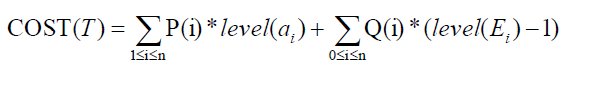把构造二分检索树的过程看成一系列决策的结果。
–决策的策略：决策树根，如果{a1,a2,…,an}存在一棵二分检索树，ak是该树的根，则内结点a1,a2,…,ak-1和外部结点E0,E1,…,Ek-1将位于根ak的左子树中，而其余的结点将位于右子树中
若：levelT(X)为结点X在树T中的级数，level(X)为X在T的左/
右子树中的级数，则：levelT(X) = level(X)+1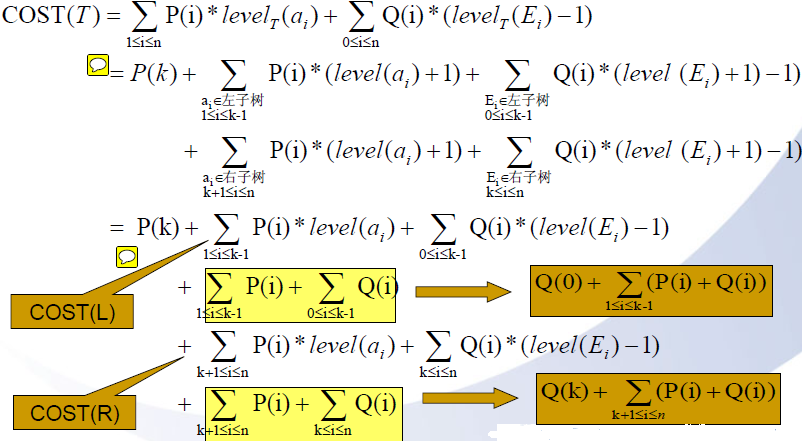目的是求出递推公式：
COST(T)与COST(L）和COST(R)之间的关系
把level(ai)+1分开，1单独拿出来了，剩下的就分别是左子树和右子树的成本（土黄色部分）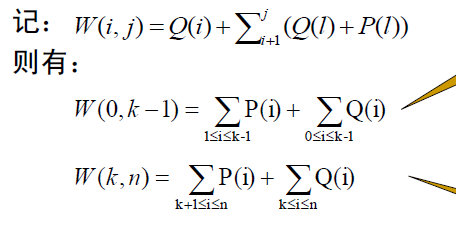W[i,j] 表示i-j所有节点的概率和，包括成功检索的概率和不成功检索的概率。
所以树的最小成本可以表示成关于左右子树的最小成本的等式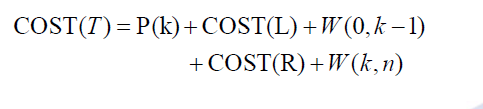解释：因为在左右子树添加一个根节点，导致左右子树的所有节点的深度增加了1，所以加上W[i,j]，这里相当于W[0,n]，
表示所有节点检索成功或者不成功的概率和。W[0,n] = P(k)+W(0,k-1)+W(k,n)。
同理，用到树的每颗子树都会成立。每科子树的最小成本设为C(i,j)，那么有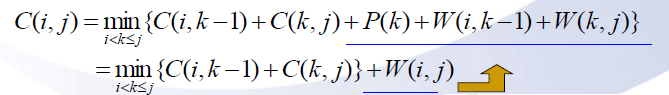C[i,j] 表示点i+1，i+2到点j中，选择任意一个点作为根，在（j-i）个解中找出成本最小的最优解

向前递推过程：
首先计算所有j-i=1的C(i, j)
然后依次计算j-i=2,3,…,n的C(i,j)。
C(0,n)=最优二分检索树的成本。

初始值
C(i,i) = 0
W(i,i) = Q(i)，0≤i≤n
最优二分检索树的构造
在计算C(i, j)的过程中，记下使之取得最小值的k值，即树Tij的根，记为R(i, j)。
依据R(0, n)…，推导树的形态

#include <iostream>

using namespace std;
#define MAX 65536
#define LENGTH 64

float W = {0};
float C = {0};
int R = {0};

int osbtree[LENGTH] = {0};
int index;
void OBST(float p[],float q[],int n)
{
int i,j,k;
float min;
for(i=0;i<=n-1;++i)
{
W[i][i] = q[i];
R[i][i] = 0;
C[i][i] = 0;
W[i][i+1] = W[i][i]+p[i+1]+q[i+1];
R[i][i+1] = i+1;
C[i][i+1] = W[i][i+1];
}
W[n][n] = q[n];
R[n][n] = 0;
C[n][n] = 0;
//m代表目前构造的树有m个节点，
//m从2到n个节点
for(int m=2;m<=n;++m)
for(i=0;i<=n-m;++i)
{
//每次比较必须重置min，否则根节点可能不改变
min = MAX;
j = i+m;
W[i][j] = W[i][j-1]+p[j]+q[j];
for(int l=i+1;l<=j;++l)
{
//
if((C[i][l-1]+C[l][j]) < min)
{
min = (C[i][l-1]+C[l][j]);
k = l;
}
}
C[i][j] = W[i][j]+C[i][k-1]+C[k][j];
R[i][j] = k;
}
}

void PrintTree(int i,int j)
{
if(i >= j)
return;
//存储根节点的标号
osbtree[index++] = R[i][j];
//左子树,存储输出左子树标志/
osbtree[index++] = '/';
PrintTree(i,R[i][j]-1);
/*右子树,存储标志输出右子树标志\*/
osbtree[index++] = '\\';
PrintTree(R[i][j],j);
}

int main()
{
char string = {"","end","goto","print","stop"};
//float p = {0,0.05,0.2,0.1,0.05};
//概率值扩大了20倍
float p = {0,1,4,2,1};
//float q = {0.2,0.1,0.2,0.05,0.05};
float q = {4,2,4,1,1};
OBST(p,q,4);
PrintTree(0,4);
/*
for(int i=0;i<5;++i)
{
for(int j=0;j<5;++j)
//打印格式i表示行坐标，j表示列坐标
cout<<W[j][j+i]<<","<<C[j][j+i]<<","<<R[j][j+i]<<'\t';
cout<<endl;
}
*/
//打印根节点
cout<<'\t'<<string[osbtree]<<endl;
for(int i=1;i<index;++i)
{
if(osbtree[i] == '/' || osbtree[i]=='\\')
continue;
else if(osbtree[i-1] == '/')
cout<<string[osbtree[i]];
else if(osbtree[i-1] == '\\')
cout<<"\t\t"<<string[osbtree[i]]<<endl;
}
return 0;
}



展开全文• 单源点最短路径，最优二分检索树算法程序实现，包含设计文档源代码
• 这个程序可实现最优二分检索树的构造，绘制检索，请在Turboc 2.0下运行。
• 下面逐步的来讲解怎么样通过动态决策构建最优二分检索树，首先一步一步的来： 前奏： 二分检索树（Binary Search Tree）的定义：二分检索树是一棵二元树，它或者为空，或者其每个结点的数据元素都可以比较大小，且...
首先总的纲领就是最优决策原理：
过程的最优决策序列具有如下性质：无论过程的初始状态和初始决策是什么，其余的决策都必须相对于初始决策所产生的状态构成一个最优决策序列。
下面逐步的来讲解怎么样通过动态决策构建最优二分检索树，首先一步一步的来：
前奏：
二分检索树（Binary Search Tree）的定义：二分检索树是一棵二元树，它或者为空，或者其每个结点的数据元素都可以比较大小，且满足下面性质：（1）T的左子树中的所有元素都比根结点中的元素小。（2）T的右子树中的所有元素都比根结点中的元素大。（3）T的左子树和右子树也是二分检索树。注：Binary Search Tree要求树中的结点元素值互异。
对于一个给定的标识符集合，可能有若干个不同的二分检索树。不同的二分检索树对标示符的检索性能是不同的。例如标志符集合{a1，a2，a3,a4,a5}=(for,if,loop,repeat,while)，如果以字典顺序定义字符串大小，则可能构建的二分检索树如下：可以看出他们成功检索的平均比较次数是不同的。二分检索树的检索性能特征：
（1）成功检索：X恰好为标识符集合中的一个元素，成功检索共有n中情况，分别代表n个标识符。每个标识符都有自己的检索频率，也就是检索概率，记为：Pi（i=1,2,...,n）。
（2）不成功检索：X不是标识符集合中的任何元素，不成功检索的情况有n+1种，同样的记不成功检索的概率为Qi（i=0,1,2,...,n）。注：不成功检索的情况包括第一个元素的左边，和最后一个元素的右边，所以有n+1中情况。
同时，如果搜索一个元素，整个事件的概率空间为：{找到了，没找到}，所以有：标识符检索所需要的次数：成功检索：等于结点的级数（或结点到根的距离+1）。不成功检索：在二分检索树中引入外部结点，外部结点作为“内部结点树”的“叶子结点”，每个外部结点代表一种不成功的检索的情况。不成功检索时比较的次数等于外部结点的级数-1（外部结点到树根的距离）。例如：(1)(2)(1)AVG成功=（1+2+2+3+4）/5=2.4    AVG不成功=（2+2+2+3+4+4）/6=2.83    AVG=[（1+2+2+3+4）+（2+2+2+3+4+4）]/11=2.64(2)略.通过上面的两棵二分检索树可以看出，不同形态的二分检索树的检索性能是不同的。所以就要构建最优二分检索树（Optimal—Binary Search Tree），使检索成本最小。二分检索树的预期成本：平均检索成本=Σ每种情况出现的概率×该情况下所需的比较次数。平均检索成本的构成：成功检索成本＋不成功检索成本。成功检索成本：ΣP(i)*level(ai)）（i=1,2,...,n）。不成功检索成本：ΣQ(i)*(level(Ei)-1)(i=0,1,2,...,n)。其中Ei是不成功检索的等价类，共有n+1个等价类Ei(0≤i≤n)。预期总的成本公式如下：求最优二分检索树的问题，就是求解一个预期成本最小的二分检索树。求解最优二分检索树的方法有两种：（1）枚举法（2）动态规划策略
正题：用动态规划策略构建一棵最优二分检索树。首先回顾一下动态规划的相关知识：动态规划是通过组合子问题的解而解决整个问题的，不同于分治算法的是动态规划适用于子问题不是独立的情况。动态规划算法对每个子子问题只求解一次，将其结果保存在一张表中。
把构造二分检索树看成一系列的决策过程，首先决策树根，如果ak是该树的根，那么内结点a1,a2,...a(k-1)和外部结点E0,E1,...E(k-1)将位于ak的左子树中；其余的结点位于右子树中。这样我们很容易计算出两个子树的根本：注意：左、右子树中所有结点的级数是相对于这棵子树的根测定。这样的话，比相对于原树的根的测定值少1。为什么这么计算？因为动态规划是把大的问题分解为性质形同的小的问题，只不过这些问题不是独立的。这样的话，每次的决策都要补左子树和右子树的成本差额这样的话原二分检索树的预期成本可以表示为：这个公式就是我们用动态规划构建最优二分检索树的基本，也就是把一个原本的二分检索树的成本，划分成了它的左子树和右子树的成本，加上一个差值。当我们从最小的子树加上来，能够使得COST(T)最小的那个树就是最优二分检索树。引入一个记号：记由ai+1,ai+2,…,aj和Ei,Ei+1,…,Ej构成的最优二分检索树的预期成本为C(i, j)，并不知道谁是根结点。如果二分检索树以ak为根，则：COST（L）=C(0,k-1)因为左子树是：a1,...,a(k-1)和E0,...E(k-1)。COST（R）=C(k,n)因为右子树是：a(k+1),...an和Ek,...En。所以：这个公式的含义就是让这n个字符轮流做根结点，最后的最优二分检索树的根就是产生最小成本的那个根结点，依次的迭代下去。其中W(0，n)=P(K)+W(0,k-1)+W(k,n)。推而广之：对于任意的i，j。我们有：那么我们用动态规划构建最优二分检索树的思想基础就构建起来了：
动态规划的最优性原理是这样的：
过程的最优决策序列有这样的性质，无论过程的初始状态和初始决策是什么，其他的决策都必须相对于初始决策所产生的状态构成一个最优决策序列。
也就是说，我们要找一个字符集的整个的过程的最优决策序列，那么这个最优决策序列一定包含了许多小的最优决策序列。说白了就是，5个字符的最优决策序列一定包含4个字符、3个字符、2个字符、1个字符的最优决策序列。
所以我们在求C(i,j)的过程中，先令j-i=1，找到一个字符的最优决策序列，然后令j-i=2，找到两个字符的最优决策序列，扩展开来就能找到n个字符的最优决策序列。求解的初始值：我们在构建最优二分检索树的时候，不仅要计算出他的最小成本，还要知道这棵最优二分检索树的形态。在计算C(i, j)的过程中，记下使之取得最小值的k值，即树Tij的根，记为R(i, j)，从而能推导出这个树的形态。
综上所述，构建一个最优二分检索树的递推公式为：实例
设n=4,且(a1,a2,a3,a4)=(do if read while)。P[1:4] = (3, 3, 1, 1)，Q[0:4] = (2, 3, 1, 1, 1)，构造最优二分检索树。
提示：初始 W(i, i)=Q(i)C(i, i)=0R(i, i)=0且有，W(i, j)=P(j)+Q(j)+W(i,j-1)
构造的结果：说明：j-i依次等于0,1,2,3,4表明先构造含有一个字符的最优二分检索树，然后依次构造含有2个字符，3个字符，4个字符的最优二分检索树。i从0变化的4表明当j-1的值固定后，也就是字符的个数确定了，依次实验不同的字符找到产生最小成本的字符段。R(i,j)表示在a(i+1)到aj的字符段中，选哪个能够产生最小成本，它用来构建最后的最优二分检索树。最后的结果：C(0,4)=32表示最优二分检索树的成本为32，通过R(i,j)构建最优二分检索树：
T04=2 =>T01(左子树），T24(右子树）（在0~4这段字符集中，第2个作为根能最优，一下解释类似）T01=1 =>T00(左子树），T11(右子树）T24=3 =>T22(左子树），T34(右子树）T34=4 =>T33(左子树），T44(右子树）所以最后的树为：在程序实现之前，分析一下它的时间复杂度：对于j-i=m的情况下，有n-m+1个C(i, j)要计算。C(i,j)的计算：O(m)。每个C(i, j)要求找出m个量中的最小值。
则，n-m+1个C(i, j)的计算时间：Ｏ(nm-m2)对所有可能的m，则总的时间复杂度为：上面看到，计算C(i,j)的时间复杂度为O(m)，引入克努特方法，最优的k∈[R(i, j-1),R(i+1, j)]。能在几乎O(1)的时间复杂度内找到k，所以C(i,j)的计算时间复杂度为O(1)。这样的话，构造最优二分检索树的时间复杂度为O(n^2)。
代码：

转载于:https://www.cnblogs.com/stemon/p/3407773.html
展开全文• 如果已知集合元素的搜索概率，那么自然会提出一个关于最优二叉搜索的问题，搜索中的平均比较数是可能的最小值。 例如，考虑四个要搜索的键a、b、cd，其概率分别为0.1、0.2、0.40.3。则0.11+0.22+0.43+0.34=2.9...

算法说明
构造最佳二叉搜索树：
如果已知集合元素的搜索概率，那么自然会提出一个关于最优二叉搜索树的问题，搜索中的平均比较数是可能的最小值。
例如，考虑四个要搜索的键a、b、c和d，其概率分别为0.1、0.2、0.4和0.3。则0.11+0.22+0.43+0.34=2.9
源代码

#include <cstdio>
#include <cstring>

#define maxn 101
#define minc(a, b) (a) > (b) ? (b) : (a)
#define INF 1 << 29

double c[maxn][maxn], p[maxn];
int n, root[maxn][maxn];
int main() {
memset(c, 0, sizeof(c));
memset(root, 0, sizeof(root));
freopen("7.optimalBinarySearchTrees.txt", "r", stdin);
scanf("%d", &n);
for(int i = 1; i <= n; i++) {
scanf("%lf", &p[i]);
}

//init
for(int i = 0; i <= n; i++) {
c[i][i-1] = 0;
c[i][i] = p[i];
root[i][i] = i;
}
for (int k = 1; k <= n; k++) {
for (int i = 1; i <= n - k + 1; i++) {
int j = i + k - 1;
double sum = 0;
c[i][j] = INF;

for(int k = i; k <= j; k++) {
sum += p[k];
}
//			printf("%d, %d, %.1f\n", i, j, sum);
for (int r = i; r <= j; r++) {
double t = c[i][r - 1] + c[r + 1][j] + sum;
if (c[i][j] > t) {
c[i][j] = t;
root[i][j] = r;
}
}
}
}

for(int i = 1; i <= n + 1; i++) {
for(int j = 0; j <= n; j++) {
if(c[i][j] == 0) printf("null ");
else printf("%-5.1f", c[i][j]);
}
printf("\n");
}

printf("\n");
for(int i = 1; i <= n + 1; i++) {
for(int j = 0; j <= n; j++) {
if(c[i][j] == 0) printf("null ");
else printf("  %-3d", root[i][j]);
}
printf("\n");
}

return 0;
}


输入数据运行结果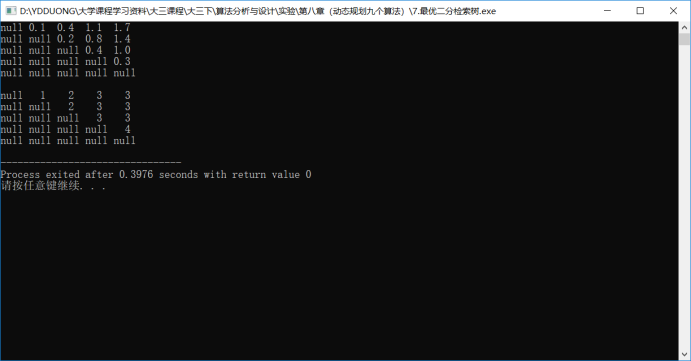展开全文动态规划
• 　(1）二叉搜索树 （二分检索树）二叉搜索树Ｔ是一棵二元树，它或者为空，或者其每个结点含有一个可以比较大小的数据元素，且有： 　a·Ｔ的左子树的所有元素比根结点中的元素小；　b·Ｔ的右子树的所有元素比根结点...
• 题目给定数据集 ...求一棵最优的( 即平均比较次数最少的)二分检索树.动态规划思路令w[i,j]是P[i,j]中所有概率(数据与空隙)之 设m[i,j] 是相对于输入S[i,j] P[i,j] 的最优二叉搜索树的平均比较次数数据
• (1）二叉查找树（二分检索树）二叉搜索树 Ｔ是一棵二元树，它或者为空，或者其每个结点含有一个可以比较大小的数据元素，且有： Ｔ的左子树的所有元素比根结点中的元素小； Ｔ的右子树的所有元素比根结点中的元素...算法
• 比如图的多起点与多终点的最短路径问题、矩阵链乘问题、最大投资效应问题、背包问题、最长公共子序列问题、图像压缩问题、最大子段问题、最优二分检索树问题等等。
• /** 动态规划(Dynamic Programming)技术广泛应用于许多组合优化问题中 e.g. 1.Floyd 2.矩阵链的乘法 3.最大效益投资 4....最优二分检索树 9.RNA的最有二级结构 关键词： 记忆花搜索 01背包问题...
• DP技术广泛应用于许多组合优化问题，比如图的多起点与多终点的最短路径问题，矩阵链的乘法问题，最大效益投资问题，背包问题，最长公共子序列问题，图像压缩问题，最大子段问题，最优二分检索问题，RNA的最优...
• kd是一种便于对k维空间中的数据进行快速检索的数据结构。kd是二叉树，表示对????k维空间的一个划分，其每个结点对应于????k维空间划分中的一个超矩形区域。利用kd可以省去对大部分数据点的搜索， 从而减少搜索
• 7.6　最优二问题 7.7　的带权完垒支配问题 7.8　的带权单步图边的搜索问题 7.9　用动态规划方法解决1螺旋多边形m守卫路由问题 7.10　实验结果 7.11　注释与参考 7.12　进一步的阅读资料 习题 第8章...算法
• 7.6　最优二问题 7.7　的带权完垒支配问题 7.8　的带权单步图边的搜索问题 7.9　用动态规划方法解决1螺旋多边形m守卫路由问题 7.10　实验结果 7.11　注释与参考 7.12　进一步的阅读资料 习题 第8章　NP完全...
• 7.6　最优二问题 7.7　的带权完垒支配问题 7.8　的带权单步图边的搜索问题 7.9　用动态规划方法解决1螺旋多边形m守卫路由问题 7.1o　实验结果 7.11　注释与参考 7.12　进一步的阅读资料 习题 第8章　np完全...
• 参考文献 第5章 特征选择 5.1 引言 5.2 预处理 5.3 峰值现象 5.4 基于统计假设检验的特征选择 5.5 接收机操作特性（ROC）曲线 5.6 类可性测量 5.7 特征子集的选择 5.8 最优特征生成 5.9 神经网络特征生成/选择 ...
• 8.2.3 二分查找 145 8.3 递归与分治 148 8.3.1 棋盘覆盖问题 148 8.3.2 循环日程表问题 149 8.3.3 巨人与鬼 149 8.3.4 非线性方程求根 150 8.3.5 最大值最小化 151 8.4 贪心法 151 8.4.1 最优装载问题 151 8.4.2 ...
• 主要用于关键信息的搜索，类似于在空间中的二分搜索，大大提高了搜索效率，在寻找目标元素时，使用了DFS深度优先的方式回溯进行最近点的寻找。详细介绍链接 MS-Apriori 基于多支持度的Apriori算法。是Apriori...
• ## 数据挖掘论文合集-242篇（part1）

千次下载 热门讨论 2009-01-13 14:03:31
文本挖掘、数据挖掘知识管理——十一世纪的智能信息处理.caj 文本数据的数据挖掘算法.caj 新的鲁棒推理控制系统设计方法.pdf 无换向器电动机在窑尾排风上的应用.pdf 最优加权系数的神经优化方法.pdf 格子机数据...
• 2.11.5 决策树和熵的联系 63 2.11.6 熵的概念及定义 63 2.11.7 理解信息增益 64 2.11.8 决策树中熵、条件熵和信息增益的联系 64 2.11.9 决策树算法中剪枝的作用及策略 65 2.12 支持向量机（SVM） 65 2.12.1 什么是...
• ## 数据挖掘论文合集-242篇（part2）

千次下载 热门讨论 2009-01-13 14:06:31
文本挖掘、数据挖掘知识管理——十一世纪的智能信息处理.caj 文本数据的数据挖掘算法.caj 新的鲁棒推理控制系统设计方法.pdf 无换向器电动机在窑尾排风上的应用.pdf 最优加权系数的神经优化方法.pdf 格子机数据...
• 文本挖掘、数据挖掘知识管理——十一世纪的智能信息处理.caj 文本数据的数据挖掘算法.caj 新的鲁棒推理控制系统设计方法.pdf 无换向器电动机在窑尾排风上的应用.pdf 最优加权系数的神经优化方法.pdf 格子机数据...
• 第十章 变法 12·1 一般概念 12·2 固定边界的变分问题 12·3 泛函极值的充分条件 12·4 可动边界的变分问题 12·5 条件变分问题 12·6 变分问题的直接法 12·7 力学中的变分原理 第十三章 概率论 13·1 基本...
• 任务：把存储、检索、共享保护文件的手段，提供给操作系统本身用户，以达到方便用户提高资源利用率的目的。 功能： ---分配与管理外存 ---提供合适的存储方法 ---文件共享、保护，解决...
• 文本挖掘、数据挖掘知识管理——十一世纪的智能信息处理.caj 数据挖掘技术在入侵检测系统中的应用.kdh 数据挖掘技术应用研究.kdh Web数据挖掘的BN实现方案.kdh Web使用模式研究中的数据挖掘.caj 数据挖掘中决策...
• ，有顶部的底部的树叶，的顶部包含着最一般的信息，例如，你要读段落顺序， 的底部是诸如一行中的词序或是一个词中的字母顺序的一些东西。 逻辑分析过程将提取这些形式信息，然后按顺序遍历此，并设法...php